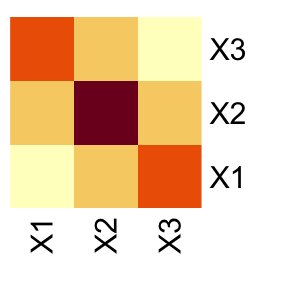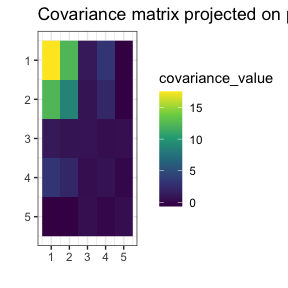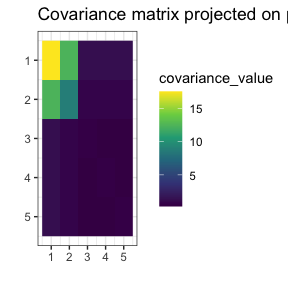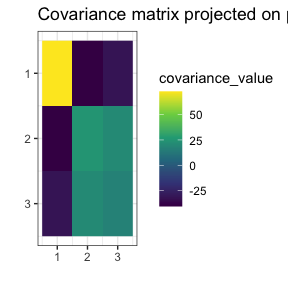# The problem

Quite often, we have too little data to perform valid inference. Consider the situation with multivariate Gaussian distribution, where we have few observations compared to the number of variables. For example, that’s the case for graphical models used in biology or medicine. In such a setting, the usual way of finding the covariance matrix (the maximum likelihood method) isn’t statistically applicable. What now?

# Invariance by permutation

In some cases, the interchange of variables in the vector does not change its distribution. In the multivariate Gaussian case, it would mean that they have the same variances and covariances with other respective variables. For instance, in the following covariance matrix, variables X1 and X3 are interchangeable, meaning that vectors (X1, X2, X3) and (X3, X2, X1) have the same distribution.Now, we can state this interchangeability property in terms of permutations. In our case, the distribution of (X1, X2, X3) is invariant by permutation ($$1\mapsto3$$, $$3\mapsto1$$), or in cyclic form $$(1,3)(2)$$. This is equivalent to saying that swapping the first with the third row and then swapping the first and third columns of the covariance matrix results in the same matrix. Then we say that this covariance matrix is invariant by permutation.

Of course, in the samples collected in the real world, no perfect equalities will be observed. Still, if the respective values in the (poorly) estimated covariance matrix were close, adopting a particular assumption about invariance by permutation would be a reasonable step.

# Package gips

We propose creating a set of constraints on the covariance matrix to use the maximum likelihood method. The constraint we consider is - none other than - invariance under permutation symmetry.

This package provides a way to find a reasonable permutation to be used as a constraint in covariance matrix estimation. In this case, reasonable means maximizing the Bayesian posterior distribution when using a Wishart-like distribution on symmetric, positive definite matrices as a prior. The idea, exact formulas, and algorithm sketch are explored in another vignette that can be accessed by vignette("Theory") or on its pkgdown page.

# Example

library(gips)

toy_example_data
#>             [,1]       [,2]     [,3]      [,4]      [,5]
#> [1,] -6.18689451 -2.9542014 5.556256 -9.036670 -6.315714
#> [2,] -2.36507452  0.2478195 6.053825 -8.661990 -6.378346
#> [3,] -0.07676498  1.3713941 7.509877 -7.587683 -5.477410
#> [4,] -9.56181675 -5.0963848 6.831322 -9.486587 -5.216622

dim(toy_example_data)
#>  4 5
number_of_observations <- nrow(toy_example_data) # 4
perm_size <- ncol(toy_example_data) # 5

S <- cov(toy_example_data)

sum(eigen(S)$values > 0.00000001) #>  3 Note that the rank of the S matrix is 3, despite the number_of_observations being 4. This is because cov() estimated the mean on every column to compute S. We want to find reasonable additional assumptions on S to make it easier to estimate. g <- gips(S = S, number_of_observations = nrow(toy_example_data)) plot(g, type = "heatmap")Looking at the plot, one can see the similarities between columns 3, 4, and 5. They have similar variance and covariance to each other. The 3 and 5 have similar covariance with columns 1 and 2. However, the 4 is not far from them. Let’s see if gips will find the relationship: g_map <- find_MAP(g, optimizer = "brute_force", return_probabilities = TRUE, save_all_perms = TRUE) #> ================================================================================ #> ================================================================================ plot(g_map, type = "heatmap")gips decided that $$(3,4,5)$$ was the most reasonable assumption. Let’s see how much better it is: g_map #> The permutation (3,4,5) #> - was found after 120 log_posteriori calculations #> - is 2.85859736142469 times more likely than the starting, () permutation. This assumption is over two times more believable than making no assumption. Let’s examine how reasonable are other possible assumptions: get_probabilities_from_gips(g_map) #> () (4,5) (3,4) (3,4,5) (3,5) #> 0.069625825644 0.046623977652 0.159093201315 0.199032201472 0.195835965731 #> (2,3) (2,3)(4,5) (2,3,4) (2,3,4,5) (2,3,5,4) #> 0.002622358777 0.006115116997 0.001372500013 0.000103873778 0.000175939275 #> (2,3,5) (2,4) (2,4,5) (2,4)(3,5) (2,4,3,5) #> 0.000406845294 0.002769223608 0.000355566299 0.006201310949 0.000075593939 #> (2,5) (2,5)(3,4) (1,2) (1,2)(4,5) (1,2)(3,4) #> 0.000787687440 0.001251472794 0.019246405857 0.034620527849 0.044691427562 #> (1,2)(3,4,5) (1,2)(3,5) (1,2,3) (1,2,3)(4,5) (1,2,3,4) #> 0.071952188406 0.071326460302 0.000349954230 0.000305491811 0.000340898117 #> (1,2,3,4,5) (1,2,3,5,4) (1,2,3,5) (1,2,4,3) (1,2,4,5,3) #> 0.000005027906 0.000329899120 0.000284223443 0.000051592498 0.000098313374 #> (1,2,4) (1,2,4,5) (1,2,4)(3,5) (1,2,4,3,5) (1,2,5,4,3) #> 0.000122291872 0.000006389866 0.000421538365 0.000017061115 0.000006581897 #> (1,2,5,3) (1,2,5,4) (1,2,5) (1,2,5,3,4) (1,2,5)(3,4) #> 0.001880101215 0.000226747805 0.000055633275 0.000064683820 0.000150899821 #> (1,3) (1,3)(4,5) (1,3,4) (1,3,4,5) (1,3,5,4) #> 0.001109438006 0.002624870878 0.000102856213 0.000002427507 0.000003707970 #> (1,3,5) (1,3)(2,4) (1,3)(2,4,5) (1,3,2,4) (1,3,5)(2,4) #> 0.000101334880 0.001837338315 0.000046442272 0.000665675993 0.000006251218 #> (1,3)(2,5) (1,3,2,5) (1,3,4)(2,5) (1,4) (1,4,5) #> 0.033370983847 0.007120827073 0.000013126080 0.000238163705 0.000027851937 #> (1,4)(3,5) (1,4,3,5) (1,4)(2,3) (1,4,5)(2,3) (1,4)(2,3,5) #> 0.000404485768 0.000002111531 0.000557941151 0.000011465761 0.000003274064 #> (1,4)(2,5) (1,4,2,5) (1,5) (1,5)(3,4) (1,5)(2,3) #> 0.000499484627 0.000126990192 0.000319607369 0.000415309790 0.010658304600 #> (1,5)(2,3,4) (1,5)(2,4) #> 0.000048290190 0.000678438561 We see that assumption $$(3,4,5)$$ is the most likely with $$19.9\%$$ posterior probability. However, the assumption $$(3,5)$$ is also reasonable, with a posterior probability of $$19.6\%$$. So it is up to us to decide which one to choose. Remember that with the assumption $$(3,5)$$, the n0 will be 4, which would be insufficient for us to estimate covariance correctly. The assumption $$(3,4,5)$$ will be just right: S_projected <- project_matrix(S, g_map[]) sum(eigen(S_projected)$values > 0.00000001)
#>  5

Now, the estimated covariance matrix is of full rank (5).

# Practical example

Let’s examine 12 books’ thick, height, and breadth data:

library(gips)

Z <-DAAG::oddbooks[,c(1,2,3)]

number_of_observations <- nrow(Z) # 12
p <- ncol(Z) # 3

S <- cov(Z)
S
#> thick    72.69697 -40.33485 -31.74242
#> height  -40.33485  25.36992  20.58576
g <- gips(S, number_of_observations, D_matrix=diag(p)) # the default D_matrix
plot(g, type = "heatmap")We can see similarities between columns 2 and 3, representing the book’s height and breadth. In particular, the covariance between [1,2] is very similar to [1,3], and the variance if  is similar to the variance of . Those are not surprising, given the interpretation of the data.

g_map <- find_MAP(g, optimizer = "brute_force",
return_probabilities = TRUE, save_all_perms = TRUE)
#> ================================================================================
#> ================================================================================

g_map
#> The permutation ()
#>  - was found after 6 log_posteriori calculations
#>  - is 1 times more likely than the starting, () permutation.
get_probabilities_from_gips(g_map)
#>                   ()                (2,3)                (1,2)
#> 0.917699644399123216 0.082300333638115772 0.000000000064309861
#>              (1,2,3)                (1,3)
#> 0.000000021892918704 0.000000000005532453

We see the search was too restrictive and did not find the permutation. We will weaken the restrictions by changing the D_matrix parameter.

g <- gips(S, number_of_observations, D_matrix=0.05*diag(p))
g_map <- find_MAP(g, optimizer = "brute_force",
return_probabilities = TRUE, save_all_perms = TRUE)
#> ================================================================================
#> ================================================================================

g_map
#> The permutation (2,3)
#>  - was found after 6 log_posteriori calculations
#>  - is 3.57966311318165 times more likely than the starting, () permutation.
get_probabilities_from_gips(g_map)
#>                  ()               (2,3)               (1,2)             (1,2,3)
#> 0.21834865211409160 0.78161461578574631 0.00000000027813589 0.00003673179839076
#>               (1,3)
#> 0.00000000002363545

find_MAP found the symmetry represented by permutation (2,3). The result depends on two input parameters, delta and D_matrix. By default they are set to 3 and diag(p), respectively.

The method is not scale-invariant and therefore we recommend to run gips for different values of D_matrix (typically, of the form C * diag(p)).

1. To learn more about the available optimizers in find_MAP() and how to use those, see vignette("Optimizers") or its pkgdown page.
2. To learn more about the math and theory behind the gips package, see vignette("Theory") or its pkgdown page.